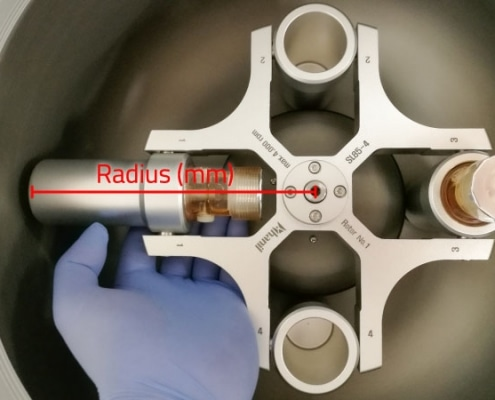## Centrifuge RPM <> RCF Conversion

The Relative Centrifugal Force (RCF)  is the amount of acceleration to be applied to the sample in the centrifuge. It depends on the Rotation Speed and the Radius of the rotor, and is relative to the force of Earth’s gravity (g-Force).

Unfortunately, many protocols and instructions only list the RPMs as a parameter for correct process setup. This is OK as long as you use only one type of rotor/centrifuge, but as soon as you try to use your process disposable on a different rotor/centrifuge, the original RPM values might be wrong if the rotor diameter differs.

Therefore, you have to convert the Rotation Speed (RPM) into Relative Centrifugation Force (RCF) and vice versa. For this purpose you will need the Rotor Radius of your centrifuge. The radius is measured from the axis of rotation to the inner bottom of the swing-out cup.

Once you have the value for the Rotor Radius “r” you can calculate RCF and/or RPM with the following formulas:Here is a tool to calculate RCF and RPM – you just need to fill in your numbers and hit Return or TAB key:
(rotor radius fields below are pre-set with the BOOST 2+ Flex (Drucker Diagnostics) rotor radius value of 139mm as mentioned in the instructions)